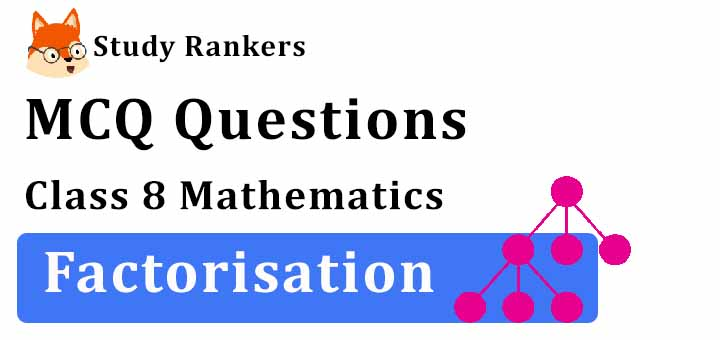>

## MCQ Questions for Class 8 Maths: Ch 14 Factorisation1. The common factor of a2 m4 and a4m2 is
(a) a4m4
(b) a2m2
(c) a2m4
(d) a4m2
► (b) a2m2

2. Divide as directed: 26xy (x + 5) (y – 4) ÷ 13x (y – 4)
(a) 2y (x + 5)
(b) (x + 5)
(c) 2y
(d) None of these
► (a) 2y (x + 5)

3. Divide as directed: 5 (2x + 1) (3x + 5) ÷ (2x + 1)
(a) 5 (3x + 5)
(b) (3x + 5)
(c) 5
(d) none of these
► (a) 5 (3x + 5)

4. Solve: –20(x)4 ÷ 10(x)2
(a) 1/2x
(b) x
(c) 1/2
(d) -2x2
► (d) -2x2

5. Find the common factors of 2y, 22xy.
(a) 2y
(b) 2
(c) 22
(d) y
► (a) 2y

6. Choose the factors of 15x2−26x+8 from the following.
(a) (3x−4),(5x+2)
(b) (3x−4),(5x−2)
(c) (3x+4),(5x−2)
(d) (3x+4),(5x+2)
► (b) (3x−4),(5x−2)

7. Find and correct the errors in the following mathematical statements. x (3x + 2) = 3x2 + 2
(a) x (3x + 2) = 3x2 + 2x
(b) x (3x + 2) = 3x2
(c) x(3x + 2) = 5x2+ 2x
(d) none of these
► (a) x (3x + 2) = 3x2 + 2x

8. Divide as directed: 52pqr (p + q) (q + r) (r + p) ÷104pq (q + r) (r + p)
(a) r(p+q)
(b) 1/2 r(p+q)
(c) 1/2
(d) none of these
► (b) 1/2 r(p+q)

9. Factorise: x2+xy+ 8x+ 8y
(a) (x + 8) (x + y)
(b) (x + y)
(c) (x + 8)
(d) (x + 9) (x – y)
► (a) (x + 8) (x + y)

10. What are the factors of x2+xy−2xz−2yz?
(a) (x−y) and (x+2z)
(b) (x+y) and (x−2z)
(c) (x−y)and (x−2z)
(d) (x+y) and (x+2z)
► (b) (x+y) and (x−2z)

11. Factorize x2 + 8x + 12
(a) (x + 2)(x + 6)
(b) (x + 3)(x + 4)
(c) 3x + 12
(d) 3x - 12
► (a) (x + 2)(x + 6)

12. How many factors does (x9−x) have?
(a) 5
(b) 4
(c) 2
(d) 9
► (a) 5

13. Which of the following is quotient obtained on dividing –18 xyz2 by –3 xz?
(a) 6 yz
(b) –6 yz
(c) 6 xy2
(d) 6 xy
► (a) 6 yz

14. Divide the given polynomial by the given monomial: (5x2− 6x) ÷ 3x
(a) (5x – 6)
(b) 1/3
(c) 1/3(5x – 6)
(d) none of these
► (c) 1/3(5x – 6)

15. Factorise 6xy – 4y + 6 – 9x.
(a) (3x – 2) (2y – 3)
(b) (3x – 2)
(c) (2y – 3)
(d) (2x – 3) (3y – 2)
► (a) (3x – 2) (2y – 3)

16. When we factorise an expression, we write it as a ________ of factors.
(a) product
(b) difference
(c) sum
(d) none of these
► (a) product

17. Factorise: 4y2 −12y + 9
(a) (7y− 5)2
(b) (5y− 3)2
(c) (2y− 5)2
(d) (2y− 3)2
► (d) (2y− 3)2

18. Amrit and Pankaj expanded (x−5)2. Amrit's answer is x2−25 and Pankaj's answer is x2−10x+25. Which of the following statements is correct?